##### Geometry: 1001 Practice Problems For Dummies (+ Free Online Practice)You can use calculus to solve practical problems, such as determining the correct size for a home-improvement project. Here’s an example. A Norman window has the shape of a semicircle above a rectangle. If the straight edges of the frame cost \$20 per linear foot and the circular frame costs \$25 per linear foot and you want a window with an area of 20 square feet, what dimensions will minimize the cost of the frame?

1. Draw a diagram and label the dimensions with variables, as shown in the following figure.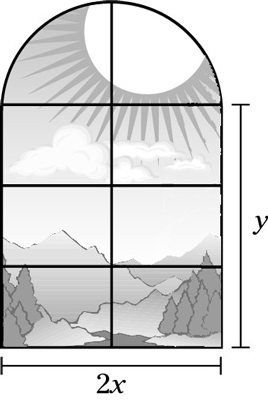2. 2Express the thing you want to minimize, the cost.

Cost = (length of curved frame) · (cost per linear foot) + (length of straight frame) · (cost per linear foot)

= (ðx)(25) + (2x + 2y)(20)

= 25ðx + 40x + 40y

3. Relate the two variables to each other.

Total Area = Area of Semicircle + Area of Rectangle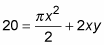4. Solve for y and substitute.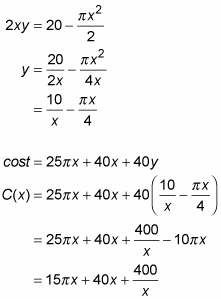5. Find the domain.

x > 0 is obvious. And when x gets large enough, the entire window of 20 square feet in area will be one big semicircle, so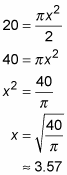Thus, x must be less than or equal to 3.57.

6. Find the critical numbers of C(x).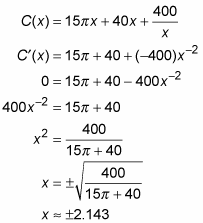Omit –2.143 because it’s outside the domain. So 2.143 is the only critical number.

7. Evaluate the cost at the critical number and at the endpoints.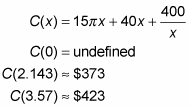So, the least expensive frame for a 20-square-foot window will cost about \$373 and will be 2 times 2.143, or about 4.286 feet or 4’3” wide at the base. Because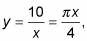the height of the rectangular lower part of the window will be 2.98, or about 3’ tall. The total height will thus be 2.98 plus 2.14, or about 5’1”.